# JP6331465B2 - Transformer connection phase determination device, transformer connection phase determination method, and transformer connection phase determination program - Google Patents

Transformer connection phase determination device, transformer connection phase determination method, and transformer connection phase determination program Download PDF

## Info

Publication number
JP6331465B2
JP6331465B2 JP2014035805A JP2014035805A JP6331465B2 JP 6331465 B2 JP6331465 B2 JP 6331465B2 JP 2014035805 A JP2014035805 A JP 2014035805A JP 2014035805 A JP2014035805 A JP 2014035805A JP 6331465 B2 JP6331465 B2 JP 6331465B2
Authority
JP
Japan
Prior art keywords
transformer
connection phase
distribution lines
likelihood
value
Prior art date
Legal status (The legal status is an assumption and is not a legal conclusion. Google has not performed a legal analysis and makes no representation as to the accuracy of the status listed.)
Active
Application number
JP2014035805A
Other languages
Japanese (ja)
Other versions
JP2015161541A (en
Inventor

Original Assignee

Priority date (The priority date is an assumption and is not a legal conclusion. Google has not performed a legal analysis and makes no representation as to the accuracy of the date listed.)
Filing date
Publication date
Application filed by 富士通株式会社 filed Critical 富士通株式会社
Priority to JP2014035805A priority Critical patent/JP6331465B2/en
Publication of JP2015161541A publication Critical patent/JP2015161541A/en
Application granted granted Critical
Publication of JP6331465B2 publication Critical patent/JP6331465B2/en
Active legal-status Critical Current
Anticipated expiration legal-status Critical

• 230000000875 corresponding Effects 0.000 claims description 83
• 238000005259 measurement Methods 0.000 claims description 29
• 238000000034 method Methods 0.000 claims description 20
• 238000004364 calculation method Methods 0.000 description 95
• 238000010586 diagram Methods 0.000 description 40
• 238000004891 communication Methods 0.000 description 6
• 230000014509 gene expression Effects 0.000 description 4
• 238000004088 simulation Methods 0.000 description 4
• 239000010432 diamond Substances 0.000 description 3
• 238000007726 management method Methods 0.000 description 3
• 230000003287 optical Effects 0.000 description 3
• 239000007787 solid Substances 0.000 description 3
• 238000001228 spectrum Methods 0.000 description 3
• 230000000576 supplementary Effects 0.000 description 3
• 230000005674 electromagnetic induction Effects 0.000 description 2
• 239000004065 semiconductor Substances 0.000 description 2
• 238000007476 Maximum Likelihood Methods 0.000 description 1
• 230000005540 biological transmission Effects 0.000 description 1
• 230000001186 cumulative Effects 0.000 description 1
• 229910003460 diamond Inorganic materials 0.000 description 1
• 238000005516 engineering process Methods 0.000 description 1
• 239000004973 liquid crystal related substance Substances 0.000 description 1
• 238000010248 power generation Methods 0.000 description 1
• 238000007781 pre-processing Methods 0.000 description 1
• 230000001105 regulatory Effects 0.000 description 1
• 239000010409 thin film Substances 0.000 description 1
• 230000001131 transforming Effects 0.000 description 1

## Images

•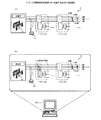•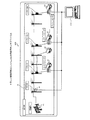•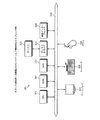•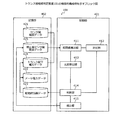•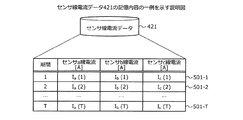•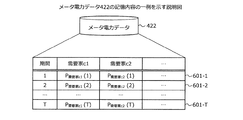•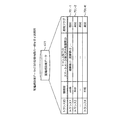•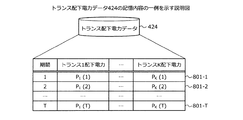•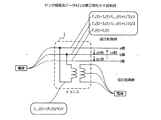•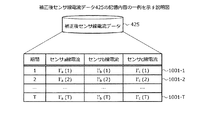•••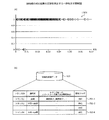•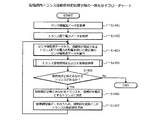•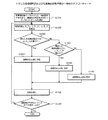•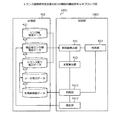•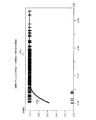•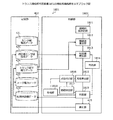•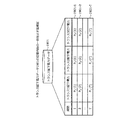•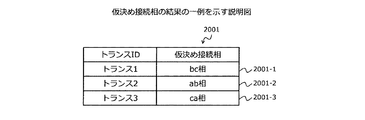•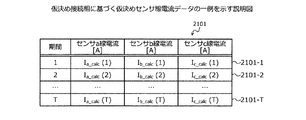•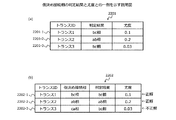•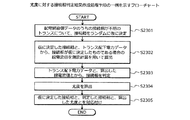## Description

The present invention relates to a transformer connection phase determination device, a transformer connection phase determination method, and a transformer connection phase determination program.
Conventionally, the connection phase of a transformer connected to any two of the three or more distribution lines may be determined in the distribution network. As a related technology, for example, the measured value of the distribution breaker is obtained via the distribution monitoring control master station and the breaker slave station, and the sensor built-in section switch is obtained via the distribution monitoring control master station and the switch slave station. There is a phase discrimination device that obtains measurement values and obtains measurement values of a smart meter via an automatic meter reading master station. In the phase determination device, distribution system configuration information, distribution transformer device information, and customer supply facility information are stored in the data storage unit. Then, the phase discrimination device selects a time section that is a period used for discrimination of the connected phase from the measurement periods of each measurement value, and stores each measurement value measured in the selected time section and the data storage unit. Based on each information, the connection phase of each distribution transformer is determined.
JP 2012-198033 A
However, according to the prior art, it is difficult to ensure the determination accuracy of the connection phase of the transformer unless information on the power consumption in all the power consumption sources connected to the transformer is obtained. For example, if there is a power consumption source that does not use a smart meter among the power consumption sources connected to the transformer, information on the power consumption at that power consumption source cannot be obtained, and the determination accuracy of the connection phase of the transformer is ensured. It becomes difficult to do.
In one aspect, an object of the present invention is to provide a transformer connection phase determination device, a transformer connection phase determination method, and a transformer connection phase determination program that improve the determination accuracy of a connection phase of a transformer.
According to one aspect of the present invention, a power value consumed by a power consumption source connected to any one of a plurality of transformers connected to any two of the three or more distribution lines, and Based on the current value flowing through each distribution line of three or more distribution lines, a correlation value indicating the correlation between power and current is calculated corresponding to each distribution line, and each calculated distribution line is supported. The two distribution lines connected to the transformer among the three or more distribution lines are determined based on the correlation value, and the determined two distribution lines are the distribution lines connected to the transformer based on the power value. The likelihood indicating the likelihood of this is calculated, and if the calculated likelihood is equal to or greater than a predetermined threshold, the current value flowing through each distribution line of the two determined distribution lines is corrected based on the power value. Transformer connection phase determination device, transformer connection phase determination method, and Connect-phase evaluation program is proposed.
According to one aspect of the present invention, it is possible to improve the determination accuracy of the connection phase of the transformer.
FIG. 1 is an explanatory diagram illustrating an operation example of the transformer connection phase determination apparatus 101. FIG. 2 is a block diagram illustrating a configuration example of the transformer connection phase determination system 200. FIG. 3 is a block diagram illustrating a hardware configuration example of the transformer connection phase determination apparatus 101. FIG. 4 is a block diagram illustrating a functional configuration example of the transformer connection phase determination apparatus 101. FIG. 5 is an explanatory diagram showing an example of the contents stored in the sensor line current data 421. FIG. 6 is an explanatory diagram showing an example of the stored contents of the meter power data 422. FIG. 7 is an explanatory diagram showing an example of the contents stored in the distribution network equipment data 423. FIG. 8 is an explanatory diagram showing an example of the storage contents of the power data 424 under the transformer. FIG. 9 is an explanatory diagram illustrating a correction example of the sensor line current data 421. FIG. 10 is an explanatory diagram showing an example of the stored contents of the corrected sensor line current data 425. FIG. 11 is an explanatory diagram of a connection phase determination example. FIG. 12 is an explanatory diagram showing a first likelihood calculation method. FIG. 13 is an explanatory diagram illustrating an example of determining correctness of a connection phase determination result. FIG. 14 is a flowchart illustrating an example of a procedure for determining a transformer connection phase in the distribution network. FIG. 15 is a flowchart illustrating an example of a transformer connection phase determination and likelihood calculation processing procedure. FIG. 16 is a block diagram illustrating a functional configuration example of the transformer connection phase determination device 1601. FIG. 17 is an explanatory diagram illustrating an example of a function created by the regression model. FIG. 18 is a block diagram illustrating a functional configuration example of the transformer connection phase determination device 1801. FIG. 19 is an explanatory diagram showing an example of the storage contents of transformer subordinate power data 1901. FIG. 20 is an explanatory diagram illustrating an example of the result of the provisional connection phase. FIG. 21 is an explanatory diagram illustrating an example of provisional sensor line current data based on the provisional connection phase. FIG. 22 is an explanatory diagram illustrating an example of a determination result and likelihood of a provisional connection phase. FIG. 23 is a flowchart illustrating an example of a connection phase determination result creation process procedure for likelihood.
Exemplary embodiments of a disclosed transformer connection phase determination device, transformer connection phase determination method, and transformer connection phase determination program will be described below in detail with reference to the drawings.
(Embodiment 1)
FIG. 1 is an explanatory diagram illustrating an operation example of the transformer connection phase determination apparatus 101. In FIG. 1, a transformer connection phase determination device 101 is a computer that determines a connection phase of a pole transformer. The pole transformer is a transformer that changes the voltage of an alternating current by the action of electromagnetic induction. In the following description, the pole transformer is simply referred to as “transformer”.
The functions required of the power transmission / distribution network, which is a means of transmitting power, have changed greatly in the current trend of regulatory reforms in the power business, including the liberalization of the power market, and the spread of renewable power. Among them, in the distribution network, it is a big problem to deal with distributed power sources such as photovoltaic power generation (Photovoltaics, PV) which are expected to be introduced in the future. As an example of the problem, it is required to manage the voltage so that the supply voltage of the distribution network falls within a certain range, for example, 101 ± 6V, 202 ± 20V.
The main problem in the voltage management of the distribution network when the power supply is concentrated in one place was the voltage drop due to overload, but in the distributed power distribution network, reverse power flow for power sale occurs Therefore, a voltage increase during reverse power flow becomes a big problem. Even in a power distribution network where power sources are concentrated in one place, design and operation have been made on the premise of preventing a voltage drop, but the problem of voltage rise is hardly assumed. In order to cope with this voltage rise problem, examination of a new voltage management system using various passive devices and active devices on power electronics has been started in various places.
Here, when examining a new voltage management method, the problem of the connection phase of a transformer surfaced. Specifically, in the three-phase three-wire distribution network, which phase is connected to the high-voltage side of the three types of distribution line combinations of the transformer at the point of contact with the power consumption source that consumes power It is a problem of not knowing. This problem can occur not only in a three-phase three-wire system but also in a distribution network using three or more wires such as a three-phase four-wire system. In the present embodiment, a three-phase three-wire distribution network will be described as an example.
Here, a combination of two distribution lines to which the transformer is connected is referred to as a “transformer connection phase”. The three types of distribution lines are referred to as “a line”, “b line”, and “c line”, respectively. A combination of the a line and the b line is referred to as “ab phase”. A combination of the b line and the c line is referred to as a “bc phase”. A combination of the c line and the a line is referred to as “ca phase”. A power consumption source that consumes power is referred to as a “customer”.
The transformer connection phase is selected so that the load of multiple consumers is balanced as much as possible in order to keep the three-phase alternating current in balance. Is difficult to manage. For this reason, when examining the application of a new voltage management method, the current state of the connection phase of the transformer is first investigated.
For example, one transformer is installed for 10 to 20 customers, and considering the entire distribution network, the number of transformers whose connection phases should be determined is enormous. Therefore, when the connection phases of all the transformers in the distribution network are manually determined, a large cost and time are consumed.
In addition, the power consumption for each phase in each distribution line section obtained the power consumption consumed by the load in each distribution line section based on the distribution line measurement value is closest to the load measurement value for each phase. Thus, it is conceivable to determine the connection phase of the transformer by obtaining the combination of the connection phases. However, since information on the power consumption of all consumers connected to the transformer is used, it is difficult to determine the connection phase of the transformer if there is a customer who does not introduce a smart meter.
Also, the connection phase is determined based on the difference in correlation between the current value measured by the sensor installed on the high-voltage line that can measure the current of each of the three-phase three-wire lines and the power value of the smart meter installed under the transformer. Can be considered. However, when the scale of the power distribution network is large, noise increases in data used for connection phase determination, and the accuracy rate of connection phase determination decreases.
Here, as a reason why the correct answer rate of the connection phase determination is reduced, for example, in order to determine the connection phase of the transformer 1, it is analyzed how a change in power under the transformer 1 changes the current measured by the sensor. It will be. However, since the power change of the customer existing under the transformer 2 also affects the current measured by the sensor, when determining the connection phase of the transformer 1, the current of the sensor that changes depending on the customer under the transformer 2 is It becomes noise and the correct answer rate of the connection phase determination is lowered.
Therefore, the transformer connection phase determination device 101 according to the first exemplary embodiment is configured so that the likelihood of the connection phase of the transformer determined from the correlation between the current flowing through each of the three or more distribution lines and the power under the transformer is equal to or greater than the threshold value. The connection current of the other transformer is determined by correcting the line current measured by the sensor. In the following description, the current flowing through the distribution line may be referred to as “line current”, and the value of the current flowing through the distribution line may be referred to as “line current value”. Thereby, the transformer connection phase determination device 101 removes noise of the line current and improves the determination accuracy of the connection phase.
FIG. 1 (a) shows a power distribution network e which is a target for studying a new voltage management method. The distribution network e is a three-phase three-wire distribution network, and includes a substation su that controls the flow of power, a sensor se, and transformers 1 and 2 as a plurality of transformers. The connection phase of transformers 1 and 2 is unknown. The sensor se measures the line current values of the a-line, b-line, and c-line, respectively.
In FIG. 1B, the transformer connection phase determination device 101 shows an example of processing for determining the connection phase of the transformers 1 and 2. With respect to the transformer 1, the transformer connection phase determination device 101 determines each of the ac lines based on the power value consumed by the consumer connected to the transformer 1 and the line current value flowing through the ac lines. Correlation values indicating the correlation between power and line current are calculated. A specific example of calculating the correlation value will be described later with reference to FIG.
Here, the power value consumed by the consumer may be a value obtained by the transformer connection phase determination device 101 obtained by a smart meter installed in the consumer, or by a user of the transformer connection phase determination device 101. It may be stored in the storage area of the transformer connection phase determination device 101. The line current value may be the current value itself measured by the sensor se, or may be a corrected current value obtained by removing noise caused by a certain transformer from the current value measured by the sensor se. Furthermore, the line current value may be a corrected current value from which noise due to power consumed under another transformer is removed from the corrected current value from which noise is removed.
Next, the transformer connection phase determination device 101 determines two distribution lines connected to the transformer 1 based on the correlation values corresponding to the lines a to c. A specific example of determining the connection phase of the transformer will be described later with reference to FIG. In the example of FIG. 1, the transformer connection phase determination device 101 determines that the connection phase of the transformer 1 is the ab phase.
Next, the transformer connection phase determination apparatus 101 calculates a likelihood indicating the likelihood of being a distribution line connected to the transformer 1, based on the power value consumed by the consumers under the transformer 1. A specific likelihood calculation example will be described later with reference to FIG. In the example of FIG. 1, the transformer connection phase determination device 101 calculates L 1 as a likelihood indicating the likelihood that the ab phase is a distribution line connected to the transformer 1.
Next, when the calculated likelihood is equal to or greater than a predetermined threshold value, the transformer connection phase determination device 101 corrects the current value flowing through each distribution line of the determined connection phase based on the power value under the transformer 1. To do. The predetermined threshold value is a threshold value specified in advance, and may be specified by a user of the transformer connection phase determination device 101, or may be determined by the transformer connection phase determination device 101. Moreover, the transformer connection phase determination apparatus 101 may calculate the likelihood of a transformer other than the transformer 1 and determine the likelihood of a transformer other than the transformer 1 as a predetermined threshold value.
In the example of FIG. 1B, it is assumed that the likelihood L 1 is equal to or greater than a predetermined threshold value. In this case, the transformer connection phase determination device 101 regards that the ab phase is the connection phase of the transformer 1 as a correct answer, and the line that has flowed through the a line and the b line based on the power value consumed by the consumer. Correct the current value. Since the line current values flowing in the corrected a-line and b-line have removed the influence of the current flowing in the transformer 1 that causes noise for transformers other than the transformer 1 in the distribution network e, the transformer connection phase determination The apparatus 101 can improve the accuracy rate of the connection phase of another transformer.
And the transformer connection phase determination apparatus 101 is based on the power value consumed by the consumer connected to the transformer 2, the current value of the corrected a line and b line, and the measured current value of the c line. A correlation value corresponding to each of the a to c lines is calculated, and the connection phase of the transformer 2 is determined.
(Configuration example of transformer connection phase determination system)
Next, a case where the transformer connection phase determination device 101 is applied to a transformer connection phase determination system that determines the connection phase of a transformer existing on the distribution network will be described.
FIG. 2 is a block diagram illustrating a configuration example of the transformer connection phase determination system 200. In FIG. 2, a transformer connection phase determination system 200 is a system for determining a connection phase of a transformer existing on the power distribution network e. The transformer connection phase determination system 200 includes a power distribution network e and a transformer connection phase determination device 101.
The distribution network e includes a substation su, a sensor se, transformers 1 to K, and consumers c1 to cn. The consumers c1 to cn include a consumer who has introduced a smart meter and a consumer who has not introduced the smart meter. For example, in FIG. 2, the consumers c1, c3, and cn have introduced smart meters, and the customer c2 has not introduced the smart meters. The smart meter is a power meter having a communication function. For example, the smart meter can grasp in real time the power usage status of homes, offices, factories, and the like that are consumers to which the smart meter is introduced.
The substation su controls the flow of electric power and sends out electric power to consumers. The transformers 1 to K are transformers that change the voltage of the alternating current by the action of electromagnetic induction. In the following description, the transformers 1 to K from the substation su in the distribution network e may be referred to as “high-voltage distribution network”. Moreover, the part from the transformers 1 to K to the consumers connected to each of the transformers 1 to K in the distribution network e may be referred to as a “low voltage distribution network”. The sensor se is installed closer to the substation su than the transformers 1 to K, and measures the current of the high-voltage distribution network. Here, in the present embodiment, the high-voltage distribution network transmits power by three-phase three-wires, that is, three electric wires, so the sensor se measures three line current values.
The transformer connection phase determination device 101 is a computer that determines the connection phases of the transformers 1 to K. Moreover, the transformer connection phase determination apparatus 101 can acquire the value which the sensor se measured the line current of ac line and the electric power value which the smart meter which the consumer introduced measured. Next, the hardware configuration of the transformer connection phase determination apparatus 101 will be described with reference to FIG.
(Hardware configuration example of transformer connection phase determination apparatus 101)
FIG. 3 is a block diagram illustrating a hardware configuration example of the transformer connection phase determination apparatus 101. In FIG. 3, the transformer connection phase determination apparatus 101 includes a CPU (Central Processing Unit) 301, a ROM (Read Only Memory) 302, and a RAM (Random Access Memory) 303. The transformer connection phase determination apparatus 101 includes a disk drive 304, a disk 305, and a communication interface 306. The transformer connection phase determination apparatus 101 includes a display 307, a keyboard 308, and a mouse 309. Further, the CPU 301 to the mouse 309 are connected by a bus 310, respectively.
The CPU 301 is an arithmetic processing device that governs overall control of the transformer connection phase determination device 101. The ROM 302 is a nonvolatile memory that stores programs such as a boot program. A RAM 303 is a volatile memory used as a work area for the CPU 301.
The disk drive 304 is a control device that controls reading and writing of data with respect to the disk 305 according to the control of the CPU 301. As the disk drive 304, for example, a magnetic disk drive, an optical disk drive, a solid state drive, or the like can be adopted.
The disk 305 is a nonvolatile memory that stores data written under the control of the disk drive 304. For example, when the disk drive 304 is a magnetic disk drive, a magnetic disk can be adopted as the disk 305. Further, when the disk drive 304 is an optical disk drive, an optical disk can be adopted as the disk 305. Further, when the disk drive 304 is a solid state drive, a semiconductor element memory, a so-called semiconductor disk, can be employed for the disk 305.
The communication interface 306 controls a network and an internal interface, and is a control device that controls data input / output from an external device. Specifically, the communication interface 306 is connected to a local area network (LAN), a wide area network (WAN), the Internet, or the like that is a network through a communication line, and is connected to another device via the network. As the communication interface 306, for example, a modem or a LAN adapter can be employed.
The display 307 is a device that displays data such as a document, an image, and function information as well as a mouse cursor, an icon, or a tool box. As the display 307, for example, a CRT (Cathode Ray Tube), a TFT (Thin Film Transistor) liquid crystal display, a plasma display, or the like can be employed.
The keyboard 308 is a device that has keys for inputting characters, numbers, various instructions, and the like and inputs data. The keyboard 308 may be a touch panel type input pad or a numeric keypad. The mouse 309 is a device for moving a mouse cursor, selecting a range, moving a window, changing a size, and the like. The mouse 309 may be a trackball or a joystick as long as it has the same function as a pointing device.
(Functional configuration example of transformer connection phase determination apparatus 101)
FIG. 4 is a block diagram illustrating a functional configuration example of the transformer connection phase determination apparatus 101. The transformer connection phase determination apparatus 101 includes a control unit 401 and a storage unit 402. The control unit 401 includes a correlation value calculation unit 411, a determination unit 412, a likelihood calculation unit 413, a determination unit 414, and a correction unit 415. The control unit 401 implements the function of the control unit 401 when the CPU 301 executes a program stored in the storage device. Specifically, the storage device is, for example, the ROM 302, the RAM 303, the disk 305, etc. shown in FIG. The processing results of each unit are stored in a register included in the CPU 301, the RAM 303, and the like.
The transformer connection phase determination apparatus 101 can access the storage unit 402. The storage unit 402 is realized by the RAM 303, the disk 305, and the like. The storage unit 402 includes sensor line current data 421, meter power data 422, distribution network equipment data 423, transformer subordinate power data 424, and corrected sensor line current data 425.
The sensor line current data 421 is a current value measured by the sensor se for each line in the distribution network e. Further, the sensor line current data 421 may be a current value for each line in a corresponding period corresponding to a period obtained by dividing a measurement period. Here, the measurement period is a period in which the power of the consumer is measured with a smart meter. An example of the stored contents of the sensor line current data 421 will be described later with reference to FIG.
The meter power data 422 is a power value obtained by measuring the power consumed by a consumer with a smart meter installed at each consumer in the distribution network e. Further, the meter power data 422 may be a power value consumed by a consumer in a corresponding period corresponding to a period obtained by dividing the measurement period. An example of the stored contents of the meter power data 422 will be described later with reference to FIG.
The distribution network facility data 423 is information regarding facilities in the distribution network e. An example of the storage contents of the distribution network facility data 423 will be described later with reference to FIG. The transformer subordinate power data 424 is data created based on the meter power data 422 and the power distribution network equipment data 423, and indicates the power consumption under each transformer. An example of the contents stored in the transformer subordinate power data 424 will be described later with reference to FIG.
The corrected sensor line current data 425 is data obtained by removing the influence of the electric power of the customer into which the smart meter under the transformer under the known connection phase is introduced on the sensor current with respect to the sensor line current data 421. . Further, the corrected sensor line current data 425 may be a current value for each line after correction in a corresponding period corresponding to a period obtained by dividing the measurement period. In FIG. 4, the sensor line current data 421 and the corrected sensor line current data 425 are shown separately. Here, the transformer connection phase determination device 101 prepares one sensor line current data 421 and generates the corrected sensor line current data 425 by updating the values of each field of the sensor line current data 421. May be. An example of the contents stored in the corrected sensor line current data 425 will be described later with reference to FIG.
The correlation value calculation unit 411 is a correlation indicating the correlation between the power and the line current corresponding to each distribution line based on the transformer subordinate power data 424 and the sensor line current data 421 or the corrected sensor line current data 425. Calculate the value. A specific calculation example will be described later with reference to FIG.
The determination unit 412 determines two distribution lines connected to the transformer among the three or more distribution lines based on the correlation value corresponding to each distribution line calculated by the correlation value calculation unit 411. A specific determination example will be described later with reference to FIG.
Based on the transformer subordinate power data 424, the likelihood calculation unit 413 calculates a likelihood indicating the likelihood that the two distribution lines determined by the determination unit 412 are distribution lines connected to the transformer. For example, the likelihood calculation unit 413 calculates the cumulative power value consumed by a consumer connected to a certain transformer or the square value of the power value consumed by a consumer connected to a certain transformer as the likelihood. The method for calculating the likelihood based on the subordinate power data 424 corresponds to a second likelihood calculating method described later with reference to FIG.
In addition, the likelihood calculating unit 413 may calculate the likelihood based on the difference between the correlation values corresponding to each distribution line. An example in which likelihood is calculated based on the difference between correlation values corresponding to each distribution line corresponds to a first likelihood calculation method described later with reference to FIG.
In addition, as a third likelihood calculation method described later in FIG. 12, the correlation value calculation unit 411 calculates a correlation value indicating the correlation between the power and the line current corresponding to each distribution line in the measurement period, and A correlation value indicating a correlation between power and line current corresponding to each distribution line in the period is calculated. Next, the determination unit 412 determines two distribution lines connected to the transformer based on the correlation value corresponding to each distribution line in the calculated measurement period, and corresponds to each distribution line in each calculated period. Two distribution lines connected to the transformer are determined based on the correlation value. And the likelihood calculation part 413 is a result in agreement with the result determined based on the correlation value corresponding to each distribution line in a measurement period among the results determined based on the correlation value corresponding to each distribution line in each period. The likelihood is calculated based on the number of.
For example, the likelihood calculation unit 413 matches the result determined based on the correlation value corresponding to each distribution line in the measurement period among the results determined based on the correlation value corresponding to each distribution line in each period. The number may be directly used as the likelihood. In addition, the likelihood calculation unit 413 matches the result determined based on the correlation value corresponding to each distribution line in the measurement period among the results determined based on the correlation value corresponding to each distribution line in each period. A value obtained by dividing the number of by the number of periods may be used as the likelihood.
The determination unit 414 determines whether the likelihood calculated by the likelihood calculation unit 413 is equal to or greater than a predetermined threshold. The threshold value specified in advance may be specified by the user of the transformer connection phase determination device 101, or may be determined by the transformer connection phase determination device 101.
When the determination unit 414 determines that the likelihood is equal to or greater than a predetermined threshold, the correction unit 415 flows to each distribution line of the two distribution lines determined by the determination unit 412 based on the transformer subordinate power data 424. Correct the current value. A specific correction example of power correction will be described later with reference to FIG. Further, the correction unit 415 stores the corrected current value in the corrected sensor line current data 425.
FIG. 5 is an explanatory diagram showing an example of the contents stored in the sensor line current data 421. The sensor line current data 421 is a current value measured by the sensor for each line in the considered distribution network.
The sensor line current data 421 includes fields of a period, a sensor a line current, a sensor b line current, and a sensor c line current. The period field indicates a period during which the sensor measures current. Here, the periods in the first to third embodiments are represented by natural numbers from 1 to T for the sake of simplification of description. Therefore, the natural number stored in the period field in the first to third embodiments is a value for identifying the period. For example, period 1 is a period from 0:00 to 0:30 on January 1. Period 2 is a period from 0:30 on January 1 to 1:00. Moreover, the period which combined the periods 1-T becomes a measurement period.
The sensor a-line current field stores a current value flowing through the a-line. The sensor b-line current field stores a current value flowing through the b-line. The sensor c-line current field stores a current value flowing through the c-line.
The sensor line current data 421 illustrated in FIG. 5 includes records 501-1 to 50-T. For example, in the record 501-1, in the period 1, the current value flowing through the a line is I a (1) [A], the current value flowing through the b line is I b (1) [A], and the c line It is shown that the current value flowing through Ic is I c (1) [A].
FIG. 6 is an explanatory diagram showing an example of the stored contents of the meter power data 422. The meter power data 422 is a power value obtained by measuring the power consumed by a consumer with a smart meter installed at each consumer in the distribution network e. Meter power data 422 shown in FIG. 6 has records 601-1 to 601-T.
The meter power data 422 has fields of a period and consumer power consumption at each consumer. A period field shows the period when the smart meter installed in each consumer measured power consumption. The consumer power consumption field in each consumer indicates the power value consumed by each consumer in the period indicated by the period field.
For example, in the record 601-1, in the period 1, the power value consumed by the customer c1 is P consumer c1 (1), and the power value consumed by the customer c2 is P consumer c2 (1). Indicates.
FIG. 7 is an explanatory diagram showing an example of the contents stored in the distribution network equipment data 423. The power distribution network equipment data 423 is information regarding the power receiving equipment of the customer in the power distribution network e. The distribution network equipment data 423 illustrated in FIG. 7 includes records 701-1 to 701 -K.
The distribution network facility data 423 includes fields of a transformer ID, a connection phase, a smart meter installed customer ID under the transformer, and a known flag. Information for identifying a transformer is stored in the transformer ID field. In the connection phase field, information for identifying the connection phase of the transformer identified by the transformer ID is stored. When the connection phase of the transformer identified by the transformer ID is unknown, an “unknown” identifier indicating that the connection phase is unknown is stored in the connection phase field. The smart meter installed customer ID field under the transformer stores information for identifying the smart meter installed customer under the transformer identified by the transformer ID. The known flag field stores a flag indicating whether or not the connection phase of the transformer identified by the transformer ID is known. Specifically, in the known flag field, one of a “known” identifier indicating that the connection phase of the transformer is known and an “unknown” identifier indicating that the connection phase of the transformer is unknown. An identifier is stored.
For example, the record 701-1 indicates that the connection phase of the transformer 1 is known and is the ab phase, and the consumers under the transformer 1 are the customer c1, the customer c2,.
FIG. 8 is an explanatory diagram showing an example of the storage contents of the power data 424 under the transformer. The transformer subordinate power data 424 is data created based on the meter power data 422 and the power distribution network equipment data 423, and indicates the power consumption under each transformer. Specifically, the transformer subordinate power data 424 is data created by accumulating the power consumption of consumers under the corresponding transformer for each transformer. The transformer subordinate power data 424 illustrated in FIG. 8 includes records 801-1 to T.
The transformer subordinate power data 424 includes fields of a period and subordinate transformer power in each transformer. In the period field, a value for identifying the period is stored. The transformer subordinate power field in each transformer stores the total power consumed by consumers under each transformer in the period specified by the value stored in the period field.
For example, record 801-1 indicates that in period 1, the subordinate power of transformer 1 is P 1 (1),..., And the subordinate power of transformer K is P K (1). Here, for example, P 1 (1) is the total of the power consumed by the customer c1 in the period 1, the power consumed by the customer c2 in the period 1, and so on.
FIG. 9 is an explanatory diagram illustrating a correction example of the sensor line current data 421. In FIG. 9, as an example of correction of the sensor line current data 421, an example in which data from which the influence of the power under the transformer whose connection phase is known is removed from the sensor line current data 421 is generated.
The transformer connection phase determination apparatus 101 refers to the known flag field of the distribution network equipment data 423 and identifies a transformer whose connection phase is known. In the distribution network equipment data 423 shown in FIG. 7, the transformer whose connection phase is known is the transformer 1, and therefore, in FIG. In FIG. 9, it is assumed that the transformer 1 is a single-phase three-wire transformer.
After identifying the transformer 1 whose connection phase is known, the transformer connection phase determination device 101 determines the value of the current that flows on the primary side of the transformer 1 by the subordinate power of the transformer whose connection phase is known as the following equation (9-1): Calculate using.
I 1_1 (t) = (P 1 (t) / V) / r (9-1)
Here, I 1_1 (t) represents a current value flowing on the primary side of the transformer 1. P 1 (t) indicates the power value under control of the transformer 1. Further, V indicates an estimated voltage value in the power distribution section corresponding to the transformer 1. For example, V is 100 [V]. R is a transformation ratio of the transformer 1. For example, if the voltage value on the primary side of the transformer 1 is 6600 [V] and the voltage value on the secondary side of the transformer 1 is 105 [V], r is r = 6600/105.
In addition, the transformer connection phase determination apparatus 101 calculates the current flowing on the primary side of the transformer 1 using the following equation (9-2) instead of the equation (9-1) in consideration of the reactive power. Also good.
I 1_1 (t) = (| P 1 (t) + jQ 1 (t) | / V) / r (9-2)
Here, Q 1 (t) is an estimated value of reactive power based on P 1 (t).
Next, the transformer connection phase determination device 101 converts the current value flowing into the transformer 1 into the current value at the sensor measurement point, and subtracts the converted value from the sensor line current data 421 to which the transformer 1 is connected. In the example of FIG. 9, since the connection phase of the transformer 1 is the ab phase, the transformer connection phase determination device 101 uses the a line, b, A value converted from the sensor line current data 421 to which the transformer 1 is connected is subtracted from the line. Since the transformer 1 is not connected to the c-line, the transformer connection phase determination apparatus 101 does not perform correction.
I ′ a (t) = I a (t) −I 1_1 (t) × (√3) / 2 (9-3)
I ′ b (t) = I b (t) −I 1_1 (t) × (√3) / 2 (9-4)
Here, I ′ a (t) and I ′ b (t) are sensor line current data 421 after correction of the a line and the b line, respectively. In the example of FIG. 9, there is one transformer whose connection phase is known. However, when there are a plurality of transformers whose connection phases are known, the transformer connection phase determination apparatus 101 determines the number of transformers whose connection phases are known. Times, the sensor line current data is corrected.
FIG. 10 is an explanatory diagram showing an example of the stored contents of the corrected sensor line current data 425. The corrected sensor line current data 425 is data obtained by removing the influence of the electric power of the customer into which the smart meter under the transformer under the known connection phase is introduced on the sensor current with respect to the sensor line current data 421. . The corrected sensor line current data 425 shown in FIG. 10 includes records 1001-1 to 100-T. Since the field of the corrected sensor line current data 425 is the same as the sensor line current data 421, the description thereof is omitted.
For example, in the record 1001-1, in the period 1, the corrected current value flowing through the a line is I ′ a (1) [A], and the corrected current value flowing through the b line is I ′ b (1). [A], and indicates that the corrected current value flowing in the c-line is I ′ c (1) [A].
FIG. 11 is an explanatory diagram of a connection phase determination example. In FIG. 11, the connection phase of the transformer 2 is determined from the corrected current values of the a, b, and c lines and the subordinate power of the transformer 2 whose connection phase is unknown.
In FIG. 11, graphs 1101-I ′ a , 1101-I ′ b , and 1101-I ′ c indicate the relationship between time and current of each of I ′ a , I ′ b , and I ′ c . Further, in FIG. 11, the relationship between the time and the power P 2, shown graphically 1101-P 2.
The transformer connection phase determination device 101 sets the connection phase of the transformer k to correlation coefficients ρ_P k _I ′ a and ρ_P k _I between I ′ a , I ′ b and I ′ c and the subordinate power P k of the transformer k. ' b and ρ_P k _I' c are calculated, and the connected phase is determined from the value having the smallest correlation. Here, k is a natural number from 1 to K. Specifically, the transformer connection phase determination device 101 determines, as a connection phase, a phase corresponding to an expression that satisfies a condition among the following expressions (11-1) to (11-3).
ρ_P k _I ′ c ≦ ρ_P k _I ′ a and ρ_P k _I ′ c ≦ ρ_P k _I ′ b (11-1)
ρ_P k _I ′ a ≦ ρ_P k _I ′ b and ρ_P k _I ′ a ≦ ρ_P k _I ′ c (11-2)
ρ_P k _I ′ b ≦ ρ_P k _I ′ c and ρ_P k _I ′ b ≦ ρ_P k _I ′ a (11-3)
When satisfy | filling (11-1) Formula, the transformer connection phase determination apparatus 101 determines a connection phase as ab phase. Moreover, when satisfy | filling (11-2) Formula, the transformer connection phase determination apparatus 101 determines a connection phase as a bc phase. Moreover, when satisfy | filling (11-3) Formula, the transformer connection phase determination apparatus 101 determines a connection phase as ca phase.
The transformer connection phase determination apparatus 101 calculates the correlation coefficient ρ_P k _I ′ x using the following equation (11-4). Here, x is any one of a, b, and c.
Here, I′avg x is an average value of I ′ x . The transformer connection phase determination apparatus 101 calculates I′avg x using the following equation (11-5). Pavg k is an average value of P k . The transformer connection phase determination apparatus 101 calculates Pavg k using the following equation (11-6).
Equation (11-4) analyzes the difference in the strength of the correlation between the total electric power P k of the customers who have installed the smart meter under the transformer k and the sensor currents I ′ a , I ′ b , I ′ c , The method of determining the connection phase is shown as a mathematical expression. The transformer connection phase determination apparatus 101 may apply preprocessing such as application of a digital filter with respect to P k , I ′ a , I ′ b , and I ′ c before executing equation (11-4). Good.
In the example of FIG. 11, the transformer connection phase determination device 101 determines that the expression (11-1) is satisfied, and determines the connection phase of the transformer 2 as the ab phase.
Next, a likelihood calculation method will be described. As the likelihood calculation method, the present embodiment shows three calculation methods: a first likelihood calculation method to a third likelihood calculation method. The first likelihood calculation method is a method of calculating the likelihood using the correlation coefficient used in the connection phase determination. The first likelihood calculation method will be described with reference to FIG. The second likelihood calculation method is a method of calculating the likelihood from the magnitude of the spectrum of the power under the transformer. The third likelihood calculation method is a method of calculating the likelihood from the relationship between the connection phase calculated using all data and the connection phase calculated using some data. The second likelihood calculation method and the third likelihood calculation method will be described after the description of the first likelihood calculation method.
(First likelihood calculation method)
FIG. 12 is an explanatory diagram showing a first likelihood calculation method. The transformer connection phase determination apparatus 101 calculates the likelihood L k of the transformer k using the equation (12-1-1).
L k = median (ρ_P k _I ′ a , ρ_P k _I ′ b , ρ_P k _I ′ c ) −min (ρ_P k _I ′ a , ρ_P k _I ′ b , ρ_P k _I ′ c ) (12-1- 1)
Here, median () is a function that outputs the median value among the values given by the arguments. Also, min () is a function that outputs the minimum value among the values given by the arguments. As the reason why the equation (12-1-1) shows the likelihood, the determination of the connection phase described in FIG. 11 is the minimum value among ρ_P k _I ′ a , ρ_P k _I ′ b , and ρ_P k _I ′ c. The connection phase is determined by Therefore, if the difference between the smallest correlation coefficient and the second smallest correlation coefficient is large, the determination result does not change even with a little noise, and it can be said that the likelihood of the connection phase determination result is large.
More specifically, in FIG. 12, the magnitude of the likelihood is shown using a graph 1201 shown in FIG. 12A and a graph 1202 shown in FIG. Both graphs 1201 and 1202 show correlation coefficients of ρ_P k _I ′ a , ρ_P k _I ′ b , and ρ_P k _I ′ c .
In the example of the graph 1201, since the difference between the minimum correlation coefficient ρ_P k _I ′ a and ρ_P k _I ′ b is large, the determination result remains the ab phase even if there is some noise. The likelihood L k increases.
On the other hand, in the example of the graph 1202, since the difference between the minimum correlation coefficient ρ_P k _I ′ a and ρ_P k _I ′ b is small, the true minimum value may be ρ_P k _I ′ b depending on the degree of noise. It may have been. Here, the true minimum value is the minimum value of the correlation coefficient that is found when infinite data is obtained. Therefore, although the determination result is the ab phase, as a result of ρ_P k _I ′ b , the true connection phase includes the possibility of being the ca phase, and thus the likelihood L k becomes small.
Moreover, the transformer connection phase determination apparatus 101 may calculate the likelihood L k of the transformer k using the following equation (12-1-2) instead of the equation (12-1-1).
L k = (max (ρ_P k _I ′ a , ρ_P k _I ′ b , ρ_P k _I ′ c ) + median (ρ_P k _I ′ a , ρ_P k _I ′ b , ρ_P k _I ′ c )) / 2−min ( ρ_P k _I 'a, ρ_P k _I' b, ρ_P k _I 'c) ... (12-1-2)
Here, max () is a function that outputs the maximum value among the values given by the arguments. (C) of FIG. 12 shows a list of determination results and likelihoods as a table 1203 for each transformer whose connection phase is unknown in the distribution network facility data 423 shown in FIG. The table 1203 includes records 1203-2,. For example, the record 1203-2 indicates that the determination result of the connection phase of the transformer 2 is the ab phase and the likelihood is 0.05. The transformer 1 whose connection phase is known does not exist in the table 1203 because the connection phase is not determined.
(Second likelihood calculation method)
As a second likelihood calculation method, the transformer connection phase determination apparatus 101 calculates the likelihood from the magnitude of the spectrum of the power under the transformer. Specifically, the transformer connection phase determination apparatus 101 uses the fact that the signal strength against noise increases and the likelihood increases when the power under the transformer is large, and the following equation (12-2-1) or The likelihood L k is calculated using the following equation (12-2-2).
Further, when a digital filter is applied when determining the connection phase, the frequency component cut by the digital filter does not affect the determination of the connection phase. Therefore, when a digital filter is applied when determining the connection phase, the accuracy can be improved by calculating the likelihood by excluding the frequency component cut by the digital filter. Specifically, transformer connection phase determination apparatus 101 calculates likelihood L k using the following equation (12-2-3).
Here, F represents a set of frequencies that are not cut by the digital filter. F (P k ) (f) is a discrete Fourier transform of P k (t). Specifically, F (P k ) (f) is expressed using the following equation (12-2-4).
(Third likelihood calculation method)
As a third likelihood calculation method, the transformer connection phase determination apparatus 101 calculates the likelihood from the relationship between the connection phase calculated using all data and the connection phase calculated using some data. Specifically, when the connection phase determined using all data is the same as the connection phase determined using another data set of the same transformer, the transformer connection phase determination device 101 has a high likelihood. Utilizing what can be said, the likelihood L k is calculated using the following equation (12-3-1).
L k = (number of times the same phase determination result as the connected phase obtained using all data in the data set) / (number of data sets) (12-3-1)
A specific example using the formula (12-3-1) will be described below. For the transformer k, the connection phase obtained using the data of the periods 1,..., T is set to phase (1 to T, k). Here, it is assumed that the transformer connection phase determination apparatus 101 obtains phase (1 to T, k) = ab.
Next, the transformer connection phase determination apparatus 101 generates n0 sets of data from the data of the measurement periods 1,. For example, when T = 10000 and n0 is 1000, the transformer connection phase determination apparatus 101 generates the following 10 sets of data.
1st set: data for the period 1-1000 2nd set: data for the period 1001-2000 ...
10th set: Data for period 9001 to 10000
Next, the transformer connection phase determination device 101 performs connection phase determination for each of the n0 sets of data, and obtains phase (1-1000, k),..., Phase (9001-10000, k). Next, the transformer connection phase determination apparatus 101 sets Num (ab) as the number determined as the ab phase among the phases (1-1000, k),..., Phase (9001-10000, k). And the transformer connection phase determination apparatus 101 calculates as follows using (12-3-1) Formula.
L k = Num (ab) / 10
After calculating the likelihood, the transformer connection phase determination apparatus 101 determines whether the determination result of the connection phase is correct using the magnitude of the likelihood. A specific determination example will be described with reference to FIG.
FIG. 13 is an explanatory diagram illustrating an example of determining correctness of a connection phase determination result. The transformer connection phase determination apparatus 101 determines the connection phase determination result as a correct answer if the likelihood is equal to or greater than the threshold value obtained from the simulation result as the determination result of the connection phase determination result. Here, the simulation performs the determination of the connection phase shown in FIG. 11 with respect to the transformer whose connection phase is known, and among the first likelihood calculation method to the third likelihood calculation method. The likelihood is calculated by either. A graph 1301 shown in FIG. 13A shows the relationship between the likelihood by simulation and the correctness of the determination result of the connection phase. The horizontal axis of the graph 1301 indicates the likelihood. The vertical axis is a dummy variable that takes 1 indicating a correct answer if the connection phase matches a known connection phase, and takes 0 indicating an incorrect answer if the connection phase does not match a known connection phase. is there. One of the points plotted with white diamonds in the graph 1301 is plotted against one transformer.
Referring to the graph 1301, it can be seen that when the likelihood is less than 0.01, the correct answer and the incorrect answer are mixed, whereas when the likelihood is 0.01 or more, only the correct answer exists. The transformer connection phase determination apparatus 101 specifies a threshold value based on each plot point of the graph 1301. For example, the transformer connection phase determination apparatus 101 may display the graph 1301 on the display 307 and specify the value received by the operation of the user of the transformer connection phase determination apparatus 101 who has viewed the graph 1301 as the threshold value. Moreover, the transformer connection phase determination apparatus 101 may specify a value obtained by adding a predetermined value to the maximum value of the likelihoods of incorrect answers as a threshold value. In the example of FIG. 13, it is assumed that the threshold value is specified as 0.02. If the likelihood is equal to or greater than the specified threshold value, transformer connection phase determination apparatus 101 updates distribution network equipment data 423 using the connection phase determination result as the correct answer. The likelihood calculation method by simulation and the likelihood calculation method for the connection phase determination result are the same.
FIG. 13B shows an example in which the distribution network equipment data 423 is updated by comparing the connection phase determination result shown in the table 1203 of FIG. 12C with the threshold = 0.02. Referring to Table 1203, since the likelihood is greater than or equal to the threshold in transformers 2 and K, transformer connection phase determination apparatus 101 sets the values of the connection phase fields in records 701-2 and K to ab phase and ca phase, respectively. Update.
The transformer connection phase determination device 101 determines the connection phase using the corrected sensor line current data 425, which is the result of correcting the sensor line current data 421 shown in FIG. The likelihood of the connection phase determination result is calculated. If there is no transformer that is newly regarded as a correct answer, the sensor line current data 421 cannot be corrected, and a transformer that can be regarded as a correct answer no longer appears.
Next, a flowchart executed by the transformer connection phase determination apparatus 101 will be described with reference to FIGS.
FIG. 14 is a flowchart illustrating an example of a procedure for determining a transformer connection phase in the distribution network. The transformer connection phase determination process in the distribution network is a process of determining the connection phase of the transformer in the distribution network e. The transformer connection phase determination apparatus 101 acquires sensor line current data 421 (step S1401). Next, the transformer connection phase determination apparatus 101 acquires transformer subordinate power data 424 (step S1402). Next, the transformer connection phase determination device 101 calculates corrected sensor line current data from the sensor line current data 421 excluding the influence of power under the transformer whose connection phase is known (step S1403). The corrected sensor line current data is stored in the corrected sensor line current data 425. In step S1403, in the second and subsequent processing, corrected sensor line current data is calculated from the sensor line current data 421, excluding the influence of the power under the transformer for which the connection phase is determined to be known. And stored in the corrected sensor line current data 425.
Next, the transformer connection phase determination apparatus 101 performs transformer connection phase determination and likelihood calculation processing (step S1404). The transformer connection phase determination and likelihood calculation processing will be described later with reference to FIG.
Next, the transformer connection phase determination apparatus 101 determines whether there is a transformer whose connection phase can be regarded as a correct answer (step S1405). When there is a transformer whose connection phase can be regarded as a correct answer (step S1405: Yes), the transformer connection phase determination device 101 determines a transformer whose connection phase is known as a transformer whose connection phase is known (step S1406). Next, the transformer connection phase determination apparatus 101 updates the connection phase of the transformer whose connection phase is known in the distribution network equipment data 423 (step S1407). Then, the transformer connection phase determination apparatus 101 proceeds to the process of step S1403.
When there is no transformer whose connection phase can be regarded as the correct answer (step S1405: No), the transformer connection phase determination apparatus 101 ends the transformer connection phase determination processing in the distribution network. By executing the transformer connection phase determination process in the distribution network, the transformer connection phase determination device 101 can determine the connection phase of the transformer by removing noise from other transformers.
FIG. 15 is a flowchart illustrating an example of a transformer connection phase determination and likelihood calculation processing procedure. The transformer connection phase determination and likelihood calculation processing is processing for determining the connection of the transformer and calculating the likelihood of the determination result. The transformer connection phase determination device 101 selects a transformer k for which the connection phase is unknown and the connection phase is not yet determined from the distribution network equipment data 423 (step S1501). Next, the transformer connection phase determination apparatus 101 calculates correlation coefficients ρ_P k _I ′ a , ρ_P k _I ′ b , and ρ_P k _I ′ c (step S1502). Then, trans-connected phase determination unit 101, among the calculated correlation coefficients, ρ_P k _I 'a to determine whether the minimum or not (step S1503). If ρ_P k _I 'a is the minimum (Step S1503: Yes), the transformer connected phase determination unit 101 determines the connection phase to bc-phase (step S1504).
On the other hand, when ρ_P k _I ′ a is not minimum (step S1503: No), the transformer connection phase determination apparatus 101 determines whether ρ_P k _I ′ b is the minimum among the calculated correlation coefficients. It is determined whether or not (step S1505). When ρ_P k _I ′ b is minimum (step S1505: Yes), the transformer connection phase determination device 101 determines the connection phase to be the ca phase (step S1506).
On the other hand, when ρ_P k _I ′ b is not the minimum (step S1505: No), the transformer connection phase determination device 101 determines the connection phase as the ab phase (step S1507).
After executing any one of steps S1504, S1506, and S1507, the transformer connection phase determination apparatus 101 calculates a likelihood (step S1508). Next, the transformer connection phase determination apparatus 101 determines whether all transformers with unknown connection phases have been selected (step S1509). If there is a transformer that has not been selected among transformers whose connection phase is unknown (step S1509: No), the transformer connection phase determination apparatus 101 proceeds to step S1502. When all the transformers whose connection phases are unknown are selected (step S1509: Yes), the transformer connection phase determination apparatus 101 ends the transformer connection phase determination and likelihood calculation processing. By executing the transformer connection phase determination and likelihood calculation processing, the transformer connection phase determination apparatus 101 can obtain the likelihood of the connection phase and the connection phase of the transformer.
As described above, according to the transformer connection phase determination device 101, if the likelihood of the connection phase of the transformer determined from the correlation between three or more line currents and the power under the transformer is equal to or greater than the threshold, the power under the transformer is calculated. Correct the line current and determine the connection phase of the other transformer. Thereby, since the transformer connection phase determination apparatus 101 can remove noise unnecessary for the determination of the connection phase of the transformer, it is possible to improve the determination accuracy of the connection phase of the transformer in the distribution network e. Moreover, the transformer connection phase determination apparatus 101 can estimate the connection phase of the transformer if there is a part of the power consumption information in the power consumption source connected to the transformer. Therefore, since the transformer connection phase determination device 101 does not have to prepare all the information on the power consumption in the power consumption source connected to the transformer, the transformer connection phase determination device 101 efficiently determines the connection phase of the transformer. be able to.
Moreover, according to the transformer connection phase determination apparatus 101, the likelihood may be calculated based on a difference between correlation values corresponding to each distribution line. The larger the difference between correlation values is, the more the connection phase determination result does not change even if noise is entered. Therefore, the transformer connection phase determination apparatus 101 has a higher likelihood of the more likely connection phase determination result. Can be output as a value.
Moreover, according to the transformer connection phase determination apparatus 101, among the results determined based on the correlation value corresponding to each distribution line in each period, the result determined based on the correlation value corresponding to each distribution line in the measurement period; The likelihood may be calculated based on the number of matching results. Thereby, the transformer connection phase determination apparatus 101 can increase the likelihood as the connection phase of the transformer in each period matches the connection phase of the transformer in the measurement period.
Moreover, the transformer connection phase determination apparatus 101 may combine the first likelihood calculation method to the third likelihood calculation method. For example, the transformer connection phase determination apparatus 101 uses the second likelihood calculation method or the third likelihood calculation method when there is no transformer in which the likelihood calculated by the first likelihood calculation method can be regarded as a correct answer. The likelihood may be calculated. Moreover, the transformer connection phase determination apparatus 101 performs each of the first likelihood calculation method to the third likelihood calculation method, and outputs each list of connection phases of the transformer determined by each likelihood calculation method. Good. The user of the transformer connection phase determination device 101 browses each list of transformer connection phases, and in each of the lists, the connection phases of transformers having a common connection phase are regarded as correct, and connections of transformers having different connection phases are connected. The phase may be confirmed manually as an incorrect answer.
(Embodiment 2)
In the transformer connection phase determination apparatus 101 according to the first embodiment, it can be understood that the greater the likelihood, the easier the transformer connection phase is to answer correctly. However, the information such as how much the likelihood is and the correct answer rate. Is not included. Therefore, in the transformer connection phase determination device according to the second exemplary embodiment, a regression model that represents the correspondence between the magnitude of likelihood and the accuracy rate is created, and a threshold value used for likelihood correctness is determined from the created regression model. In addition, about the location similar to the location demonstrated in Embodiment 1, the same code | symbol is attached | subjected and illustration and description are abbreviate | omitted.
(Functional configuration example of transformer connection phase determination device 1601)
FIG. 16 is a block diagram illustrating a functional configuration example of the transformer connection phase determination device 1601. In FIG. 16, the transformer connection phase determination device 1601 includes a control unit 1602 and a storage unit 402. Control unit 1602 includes a correlation value calculation unit 411, a determination unit 412, a likelihood calculation unit 413, a determination unit 1611, and a correction unit 1612. The control unit 1602 implements the function of the control unit 1602 by causing the CPU 301 to execute a program stored in the storage device. Specifically, the storage device is, for example, the ROM 302, the RAM 303, the disk 305, etc. shown in FIG. The processing results of each unit are stored in a register included in the CPU 301, the RAM 303, and the like.
The determination unit 1611 determines whether or not a probability value obtained by inputting the calculated likelihood into a function shown below is a predetermined value or more. Here, the function uses the likelihood indicating the likelihood that any two of the three or more distribution lines are distribution lines connected to the transformer. It is a function showing the probability of being a connected distribution line. Further, the aforementioned function is created by a regression model. An example of a function created by the regression model will be described later with reference to FIG.
When the determination unit 1611 determines that the probability value obtained by inputting the likelihood to the above-described function is equal to or greater than a predetermined value, the correction unit 1612 determines the 2 determined by the determination unit 412 based on the transformer subordinate power data 424. The value of the line current flowing through each distribution line of the two distribution lines is corrected.
FIG. 17 is an explanatory diagram illustrating an example of a function created by the regression model. The transformer connection phase determination device 1601 creates a regression model and determines a threshold value. In the second embodiment, the regression model is obtained by a logistic model. The logistic model is given by the following equation (17-1).
Here, L indicates likelihood. Moreover, y shows a correct answer rate. Β 0 and β 1 are parameters estimated by the maximum likelihood method or the like. Further, ε is a random variable indicating an error. With respect to the logistic model shown by the equation (17-1), the likelihood of the transformer, which is known to determine the connection phase, plotted with a diamond shape in the graph 1301 shown in FIG. A solid line 1702 in the graph 1701 shows a function generated by estimating β 0 and β 1 from the graph.
A graph 1701 is a graph representing the relationship between the likelihood and the accuracy rate of the determination result. The horizontal axis of the graph 1701 indicates the likelihood. The vertical axis of the graph 1701 indicates the accuracy rate. In addition, the points plotted in the graph 1701 indicate those plotted with white diamonds in the graph 1301.
When the logistic model is used, the user of the transformer connection phase determination device 1601 designates a predetermined value as a correct answer in advance. In the example of FIG. 17, it is assumed that the predetermined value is specified as 0.99. The transformer connection phase determination device 1601 substitutes the likelihood calculated by the method described in FIG. 12 for L with respect to the equation (17-1) in which β 0 and β 1 are estimated, and the obtained value is y = If it is 0.99 or more, the determination result of the connection phase is regarded as correct.
As described above, according to the transformer connection phase determination device 1601, the line current value is corrected if the probability value obtained by inputting the calculated likelihood into the function created by the logistic model is equal to or greater than a predetermined value. May be. The predetermined threshold in the first embodiment is specified according to the likelihood. Here, the likelihood varies greatly depending on the likelihood calculation method, and the user of the transformer connection phase determination apparatus 101 determines a predetermined threshold according to the likelihood. It is difficult to specify a threshold value. For example, the second likelihood calculation method is a method of calculating the likelihood from the magnitude of the spectrum of the power under the transformer, but the value that the likelihood can take varies greatly depending on the magnitude of the power under the transformer. . In addition, when using a plurality of likelihood calculation methods from the first likelihood calculation method to the third likelihood calculation method, the predetermined threshold varies depending on each likelihood calculation method. The user of the apparatus 101 determines a predetermined threshold value for each likelihood calculation method to be used.
On the other hand, since the probability value is between 0 and 1, the user of the transformer connection phase determination device 1601 can more easily specify the predetermined value than the predetermined threshold value. Also, when using a plurality of likelihood calculation methods among the first likelihood calculation method to the third likelihood calculation method, the user of the transformer connection phase determination device 1601 designates one predetermined value. do it.
(Embodiment 3)
The transformer connection phase determination apparatus 101 according to the first exemplary embodiment regards a correct answer if the likelihood is equal to or greater than a predetermined threshold value. Here, the specified threshold value is specified based on each plot point of the graph 1301. Here, when there are few transformers whose correct answers of the connected phases are known, the number of plotted points decreases. Therefore, for example, when the threshold value is specified by the user of the transformer connection phase determination apparatus 101, the density of the plot points of the graph 1301 becomes coarse, so that it is difficult for the user of the transformer connection phase determination apparatus 101 to specify the threshold value. . In addition, for example, when a value obtained by adding a predetermined value to the maximum value of the likelihood of being an incorrect answer is set as a threshold value, there is a high possibility of determining that the answer is correct despite being an incorrect answer. The accuracy of determining the correct answer rate decreases.
Also, the transformer connection phase determination device 1601 according to the second embodiment generates a function from the likelihood of the transformer whose connection phase determination is known and the dummy variable. Therefore, when there are few transformers whose connection phase has a known correct answer, the accuracy of determining the correct answer rate in the generated function is lowered. Thus, the transformer connection phase determination apparatus according to the third embodiment increases the number of transformers whose connection phase is known correctly. In addition, about the location similar to the location demonstrated in Embodiment 1, the same code | symbol is attached | subjected and illustration and description are abbreviate | omitted.
(Functional configuration example of transformer connection phase determination device 1801)
FIG. 18 is a block diagram illustrating a functional configuration example of the transformer connection phase determination device 1801. A transformer connection phase determination device 1801 according to the third embodiment includes a control unit 1802 and a storage unit 402. The control unit 1802 includes a correction unit 415, a connection phase provisional determination unit 1811, a line current calculation unit 1812, a correlation value calculation unit 1813, a determination unit 1814, a likelihood calculation unit 1815, an association unit 1816, A threshold value determination unit 1817, a creation unit 1818, and a determination unit 1819 are included. The control unit 1802 implements the function of the control unit 1802 when the CPU 301 executes a program stored in the storage device. Specifically, the storage device is, for example, the ROM 302, the RAM 303, the disk 305, etc. shown in FIG. The processing results of each unit are stored in a register included in the CPU 301, the RAM 303, and the like.
The connection phase provisional determination unit 1811 randomly determines two distribution lines connected to each of the three or more distribution lines, corresponding to each of the plurality of transformers. An example of randomly determining two distribution lines is shown in FIG.
The line current calculation unit 1812 is configured such that two distribution lines corresponding to each transformer are connected to each transformer based on the power value in each transformer and the two distribution lines corresponding to each determined transformer. The value of the line current flowing through each distribution line is calculated. The power value in each transformer is the power value consumed by the power consumption source connected to each transformer. Specifically, the line current calculation unit 1812 calculates a line current value by power flow calculation. An example of the result of calculating the line current value by power flow calculation is shown in FIG.
Correlation value calculation section 1813 is a correlation value corresponding to each distribution line in each transformer based on the power value in each transformer and the line current value flowing in each distribution line calculated by line current calculation section 1812. Is calculated. The calculation method of the correlation value is substantially the same, except that the current value that is an argument when the correlation value calculation unit 1813 calculates is replaced with the line current value flowing through each distribution line calculated by the line current calculation unit 1812. is there.
The determination unit 1814 determines two distribution lines connected to each transformer of the three or more distribution lines based on the correlation value corresponding to each distribution line calculated by the correlation value calculation unit 1813. The specific determination method is substantially the same, except that the correlation value at the time of determination by the determination unit 1814 is replaced with the correlation value corresponding to each distribution line calculated by the correlation value calculation unit 1813.
The likelihood calculating unit 1815 calculates a likelihood indicating the likelihood that the two distribution lines determined by the determination unit 1814 are distribution lines connected to the respective transformers, based on the power value in each transformer. . The likelihood calculation example may be any of the first likelihood calculation method to the third likelihood calculation method shown in FIG.
The associating unit 1816 includes two distribution lines corresponding to each transformer determined by the connection phase provisional determination unit 1811, two distribution lines connected to each transformer determined by the determination unit 1814, and a likelihood calculation unit. The likelihood in each transformer calculated in 1815 is output in association with it. An example of the association is shown in FIG.
The threshold determination unit 1817 compares the two distribution lines corresponding to each transformer determined by the connection phase provisional determination unit 1811 and the two distribution lines connected to each transformer determined by the determination unit 1814. Then, the threshold determination unit 1817 determines a predetermined threshold based on the comparison result compared and the likelihood in each transformer calculated by the likelihood calculation unit 1815.
For example, it is assumed that the comparison results between the two distribution lines corresponding to each transformer determined by the connection phase provisional determination unit 1811 and the two distribution lines connected to each transformer determined by the determination unit 1814 indicate coincidence. At this time, the threshold determining unit 1817 determines the predetermined threshold as the likelihood in each transformer calculated by the likelihood calculating unit 1815.
In addition, the comparison result between the two distribution lines corresponding to each transformer determined by the connection phase provisional determination unit 1811 and the two distribution lines connected to each transformer determined by the determination unit 1814 did not match. Suppose that At this time, the threshold determination unit 1817 determines the predetermined threshold to be a value larger than the likelihood in each transformer calculated by the likelihood calculation unit 1815.
The transformer connection phase determination device 1801 may include a creation unit 1818 instead of the threshold value determination unit 1817. The creation unit 1818 compares the two distribution lines corresponding to each transformer determined by the connection phase provisional determination unit 1811 and the two distribution lines connected to each transformer determined by the determination unit 1814. Then, the creating unit 1818 creates a function based on the comparison result and the likelihood in each transformer calculated by the likelihood calculating unit 1815. The function is that two distribution lines are connected to the above-described transformer using a likelihood indicating the likelihood that any two of the three or more distribution lines are distribution lines connected to the transformer. It is a function representing the probability of being a distribution line.
Hereinafter, a specific example of the third embodiment will be described. In the following description, for the sake of simplification, it is assumed that the distribution network e includes three transformers 1, 2, and 3, where K = 3. The connection phases of the three transformers are unknown. Further, consumers c1 to c10 are connected under the transformer 1, consumers c11 to c20 are connected under the transformer 2, and consumers c21 to c30 are connected under the transformer 3. Thus, it is assumed that there are 10 customers in each of the transformers 1 to 3. Suppose that 5 out of 10 houses have installed smart meters. FIG. 19 shows an example of the storage contents of the power data under the transformer when the above assumption is followed.
FIG. 19 is an explanatory diagram showing an example of the storage contents of transformer subordinate power data 1901. The transformer subordinate power data 1901 has a period field and a transformer 1 subordinate power field to a transformer 3 subordinate power field. In the period field and the transformer 1 subordinate power field to the transformer 3 subordinate power field, information having the same content as the field having the same name as the transformer subordinate power data 424 shown in FIG. 8 is stored. Moreover, the transformer subordinate power data 1901 includes records 1901-1 to 19-T.
For example, in the record 1901-1, in the period 1, the subordinate power of the transformer 1 is P 1 (1), the subordinate power of the transformer 2 is P 2 (1), and the subordinate power of the transformer 3 is P 3 (1). ).
First, the transformer connection phase determination device 1801 provisionally determines the connection phases of the transformers 1 to 3 at random. FIG. 20 shows a list of temporarily determined connection phase results.
FIG. 20 is an explanatory diagram illustrating an example of the result of the provisional connection phase. A table 2001 shows a list of connection phases provisionally determined for each transformer. A table 2001 illustrated in FIG. 20 includes records 2001-1 to 2001. For example, record 2001-1 indicates that the provisional connection phase of transformer 1 is the bc phase.
Next, the transformer connection phase determination device 1801 measures the sensor line current measured when the connection phase is provisionally determined based on the provisional connection phase and the power data 1901 under the transformer or standard power data. Calculate the data. Specifically, the transformer connection phase determination device 1801 determines the power consumed by the consumers c1 to c30. The transformer connection phase determination device 1801 uses the meter power data 422 shown in FIG. 6 as it is for the power consumed by the consumer who has already installed the smart meter among the consumers c1 to c30. On the other hand, the transformer connection phase determination device 1801 may use a general power demand model for power consumed by a consumer who has not introduced the smart meter, or exists in a distribution network different from the distribution network e. You may use the meter electric power data 422 of the customer who has introduced the smart meter.
Next, the transformer connection phase determination device 1801 obtains a current value at a point where the sensor se is installed by a circuit calculation called power flow calculation based on the power values of the consumers c1 to c30 and the impedance information of the distribution lines. . A specific example of tidal current calculation is described in Reference Document 1 below, for example. FIG. 21 shows an example of the obtained current value.
(Reference 1: MATLAB sample model manual, [searched on January 29, 2014], Internet <URL: http://www.mathworks.com/tagteam/58296_56709_TA063_Current_analysis_in_electric_power_system_paste_system_power_system_system_power_system_system_system_power_system_system_power_system_system_system_power_system_system_power_system_system_power_system_system_power_system_system_power_system_system_power_system_system_power_system_system_power_system_system_power_system_system_power_system_system_power_system_system_power_system_system_power_system_power_system_power_system_power_system_power_system_power_system_power_system_power_system_power_system_power_system_power_system_power.
FIG. 21 is an explanatory diagram illustrating an example of provisional sensor line current data based on the provisional connection phase. A table 2101 shown in FIG. 21 shows an example of provisional sensor line current data based on the provisional connection phase. Table 2101 shows a list of line current values flowing through the distribution lines in the period. A table 2101 illustrated in FIG. 21 includes records 2101-1 to 2101-1. For example, in the record 2101-1, in the period 1, the current value flowing through the a line is I a_calc (1), the current value flowing through the b line is I b_calc (1), and the current value flowing through the c line is I c_calc (1).
Next, the transformer connection phase determination device 1801 determines the connection phase based on the transformer subordinate power data 1901 shown in FIG. 19 and the provisional sensor line current data shown in FIG. Calculate the degree. The connection phase determination method is the method described in FIG. Also, the connection phase likelihood calculation method may be any of the first likelihood calculation method to the third likelihood calculation method. FIG. 22 shows an example of the determination result of the provisional connection phase and the likelihood.
FIG. 22 is an explanatory diagram illustrating an example of a determination result and likelihood of a provisional connection phase. A table 2201 shown in (a) of FIG. 22 shows the determination result and likelihood of the provisional connection phase for each transformer. The table 2201 has records 2201-1 to 2201-1. For example, the record 2201-1 indicates that the connection phase determination result of the transformer 1 is the bc phase and the likelihood is 0.1.
A table 2202 shown in FIG. 22B shows a result of associating the provisional connection phase, the determination result of the provisional connection phase, and the likelihood for each transformer. The table 2202 has records 2202-1 to 2202-1. For example, the record 2202-1 indicates that the provisional connection phase of the transformer 1 is the bc phase, the determination result is the bc phase, and the likelihood is 0.1. Accordingly, the transformer connection phase determination device 1801 determines that the determination result is correct because the provisional connection phase matches the determination result in the transformer 1. Similarly, the transformer connection phase determination device 1801 determines that the determination result is correct because the provisional connection phase matches the determination result in the transformer 2, and also determines that the determination result is the temporary connection phase in the transformer 3. And the determination result is determined to be incorrect.
Thus, by performing the processing described in FIGS. 19 to 22, it is possible to increase the points that can be plotted on the graph 1301. In the example of FIGS. 19 to 22, three points can be added. Next, a flowchart for performing the processing described in FIGS. 19 to 22 will be described with reference to FIG.
FIG. 23 is a flowchart illustrating an example of a connection phase determination result creation process procedure for likelihood. The connection phase determination result creation process for the likelihood is a process for creating a connection phase determination result for the likelihood. The transformer connection phase determination device 1801 tentatively determines a connection phase at random for a transformer whose connection phase is unknown in the distribution network equipment data 423 (step S2301). Next, the transformer connection phase determination device 1801 calculates a line current value when the connection phase is temporarily determined from the provisionally determined connection phase and the transformer subordinate power data 1901 using the power flow calculation (step S2302). ). Next, the transformer connection phase determination device 1801 determines the connection phase from the transformer subordinate power data 1901 and the calculated line current value (step S2303). For the processing in step S2303, the transformer connection phase determination device 1801 calculates a correlation value from the transformer subordinate power data 1901 and the calculated line current value. Then, the transformer connection phase determination device 1801 determines the connection phase using the correlation value.
Next, the transformer connection phase determination device 1801 calculates the likelihood (step S2304). Subsequently, the transformer connection phase determination device 1801 associates the temporarily determined connection phase, the determined connection phase, and the calculated likelihood (step S2305).
After the process of step S2305 is completed, the transformer connection phase determination device 1801 may output the temporarily determined connection phase, the determined connection phase, and the calculated likelihood. Alternatively, the transformer connection phase determination device 1801 determines whether or not the determination result is correct from the temporarily determined connection phase and the determined connection phase, and determines from the determination result and the calculated likelihood. The threshold value may be determined. When determining the predetermined threshold, the transformer connection phase determination device 1801 uses the determination result, the calculated likelihood, and the determination result of the transformer with the known connection phase shown in FIG. May be determined. Alternatively, the transformer connection phase determination device 1801 determines whether or not the determination result is correct from the temporarily determined connection phase and the determined connection phase, and determines whether the determination result is correct and uses the determination result and the calculated likelihood. A function may be created by a model. When creating the function, the transformer connection phase determination device 1801 creates a function using the determination result, the calculated likelihood, and the determination result of the transformer whose connection phase is known as shown in FIG. Also good.
After the process of step S2305 is completed, the transformer connection phase determination device 1801 ends the connection phase determination result creation process for the likelihood. By executing the connection phase determination result creation process for the likelihood, the transformer connection phase determination device 1801 can increase the connection phase determination result for the likelihood even if the connection phase determination result for the likelihood is small. A predetermined threshold can be determined, and a highly accurate function can be created.
As described above, according to the transformer connection phase determination device 1801, the line current value is calculated from the temporarily determined connection phase, and the connection phase determination and likelihood are calculated by the method described in the first embodiment. Thus, the temporarily determined connection phase, the determined connection phase, and the calculated likelihood are associated with each other. As a result, the user of the transformer connection phase determination device 1801 obtains the temporarily determined connection phase, the determined connection phase, and the calculated likelihood even if there are few transformers whose connection phase is known correctly. A predetermined threshold value can be designated with reference.
Further, according to the transformer connection phase determination device 1801, it is determined whether or not the determination result is correct from the temporarily determined connection phase and the determined connection phase, and the determination result and the calculated likelihood are used. A predetermined threshold value may be determined. Thereby, the transformer connection phase determination device 1801 can determine an appropriate predetermined threshold from the determination result and the calculated likelihood even if there are few transformers whose connection phase is correct.
Further, according to the transformer connection phase determination device 1801, it is determined whether or not the determination result is correct from the temporarily determined connection phase and the determined connection phase, and the determination result and the calculated likelihood are used. A function may be created by a logistic model. Thereby, the transformer connection phase determination device 1801 can create a highly accurate function from the determination result and the calculated likelihood even if there are few transformers whose connection phase is known correctly.
The transformer connection phase determination method described in the present embodiment can be realized by executing a program prepared in advance on a computer such as a personal computer or a workstation. The transformer connection phase determination program is recorded on a computer-readable recording medium such as a hard disk, a flexible disk, a CD-ROM (Compact Disc-Read Only Memory), a DVD (Digital Versatile Disk), and is read from the recording medium by the computer. To be executed. The transformer connection phase determination program may be distributed through a network such as the Internet.
The following additional notes are disclosed with respect to the above-described first to third embodiments.
(Supplementary note 1) The power value consumed by the power consumption source connected to any one of the plurality of transformers connected to any two of the three or more distribution lines, and the three or more distribution lines Based on the current value flowing through each distribution line of the electric wire, the correlation value indicating the correlation between the electric power and the current corresponding to each distribution line is calculated,
Based on the calculated correlation value corresponding to each distribution line, determine two distribution lines connected to the transformer among the three or more distribution lines,
Based on the power value, calculate a likelihood indicating the likelihood that the determined two distribution lines are distribution lines connected to the transformer,
If the calculated likelihood is equal to or greater than a predetermined threshold, based on the power value, the current value that has flowed through each of the determined distribution lines is corrected.
A transformer connection phase determination device comprising a control unit.
(Appendix 2) The control unit
The transformer connection phase determination device according to appendix 1, wherein the likelihood is calculated based on a difference between correlation values corresponding to each of the distribution lines.
(Appendix 3) The control unit
While calculating a correlation value indicating a correlation between power and current corresponding to each distribution line in the measurement period in which the power is measured, and power corresponding to each distribution line in each period dividing the measurement period Calculate the correlation value indicating the correlation with the current,
The two distribution lines connected to the transformer are determined based on the correlation value corresponding to each distribution line in the calculated measurement period, and the correlation value corresponding to each distribution line in the calculated period is determined. Based on the two distribution lines connected to the transformer,
Of the results determined based on the correlation value corresponding to each distribution line in each period, based on the number of results that match the result determined based on the correlation value corresponding to each distribution line in the measurement period The transformer connection phase determination device according to appendix 1 or 2, wherein the likelihood is calculated.
(Appendix 4) The control unit
Distribution line in which the two distribution lines are connected to the transformer using a likelihood indicating the likelihood that any two distribution lines of the three or more distribution lines are distribution lines connected to the transformer If the probability value obtained by inputting the calculated likelihood to a function representing the probability is greater than or equal to a predetermined value, it flows to each distribution line of the determined two distribution lines based on the power value. The transformer connection phase determination device according to any one of appendices 1 to 3, wherein the current value is corrected.
(Supplementary Note 5) The control unit
Corresponding to each transformer of the plurality of transformers, randomly determine two distribution lines connected to each of the three or more distribution lines,
Two distribution lines corresponding to each of the determined transformers based on the power value consumed by the power consumption source connected to each of the transformers and the two distribution lines corresponding to the determined transformers Calculates the value of the current flowing through each distribution line when connected to each transformer,
Based on the power value in each transformer and the calculated current value flowing in each distribution line, a correlation value corresponding to each distribution line in each transformer is calculated,
Based on the calculated correlation value corresponding to each of the distribution lines, two distribution lines connected to each of the three or more distribution lines are determined,
Based on the power value in each of the transformers, calculate a likelihood indicating the likelihood that the determined two distribution lines are distribution lines connected to each of the transformers,
The two distribution lines corresponding to the determined transformers, the two distribution lines connected to the determined transformers, and the calculated likelihoods of the transformers are output in association with each other. The transformer connection phase determination device according to any one of Supplementary notes 1 to 4.
(Appendix 6) The control unit
Compare the two distribution lines corresponding to each determined transformer and the two distribution lines connected to each determined transformer,
6. The transformer connection phase determination apparatus according to appendix 5, wherein the predetermined threshold is determined based on the comparison result obtained by comparison and the calculated likelihood in each of the transformers.
(Appendix 7) The control unit
Compare the two distribution lines corresponding to each determined transformer and the two distribution lines connected to each determined transformer,
Based on the comparison result and the calculated likelihood in each of the transformers, the likelihood that any two of the three or more distribution lines are distribution lines connected to the transformer is calculated. 6. The transformer connection phase determination apparatus according to appendix 5, wherein a function representing a probability that the two distribution lines are distribution lines connected to the transformer is created using the likelihood shown.
(Appendix 8) The computer
A power value consumed by a power consumption source connected to any one of a plurality of transformers connected to any two of the three or more distribution lines, and each of the three or more distribution lines Based on the current value flowing through the distribution line, a correlation value indicating the correlation between power and current corresponding to each distribution line is calculated,
Based on the calculated correlation value corresponding to each distribution line, determine two distribution lines connected to the transformer among the three or more distribution lines,
Based on the power value, calculate a likelihood indicating the likelihood that the determined two distribution lines are distribution lines connected to the transformer,
If the calculated likelihood is equal to or greater than a predetermined threshold, based on the power value, the current value that has flowed through each of the determined distribution lines is corrected.
A transformer connection phase determination method characterized by executing processing.
(Appendix 9)
A power value consumed by a power consumption source connected to any one of a plurality of transformers connected to any two of the three or more distribution lines, and each of the three or more distribution lines Based on the current value flowing through the distribution line, a correlation value indicating the correlation between power and current corresponding to each distribution line is calculated,
Based on the calculated correlation value corresponding to each distribution line, determine two distribution lines connected to the transformer among the three or more distribution lines,
Based on the power value, calculate a likelihood indicating the likelihood that the determined two distribution lines are distribution lines connected to the transformer,
If the calculated likelihood is equal to or greater than a predetermined threshold, based on the power value, the current value that has flowed through each of the determined distribution lines is corrected.
A transformer connection phase determination program characterized in that a process is executed.
101, 1601, 1801 Transformer connection phase determination device 401, 1602, 1802 Control unit 402 Storage unit 411, 1813 Correlation value calculation unit 412, 1814 Determination unit 413, 1815 Likelihood calculation unit 414, 1611, 1819 Judgment unit 415, 1612 Correction Unit 421 sensor line current data 422 meter power data 423 distribution network facility data 424 transformer subordinate power data 425 corrected sensor line current data 1811 connection phase provisional determination unit 1812 line current calculation unit 1816 association unit 1817 threshold determination unit 1818 creation unit

## Claims (9)

1. A power value consumed by a power consumption source connected to any one of a plurality of transformers connected to any two of the three or more distribution lines, and each of the three or more distribution lines Based on the current value flowing through the distribution line, a correlation value indicating the correlation between power and current corresponding to each distribution line is calculated,
Based on the calculated correlation value corresponding to each distribution line, determine two distribution lines connected to the transformer among the three or more distribution lines,
Based on the power value, calculate a likelihood indicating the likelihood that the determined two distribution lines are distribution lines connected to the transformer,
If the calculated likelihood is equal to or greater than a predetermined threshold, based on the power value, the current value that has flowed through each of the determined distribution lines is corrected.
A transformer connection phase determination device comprising a control unit.
2.   A power value consumed by a power consumption source connected to any one of a plurality of transformers connected to any two of the three or more distribution lines, and each of the three or more distribution lines Based on the current value flowing through the distribution line, a correlation value indicating the correlation between power and current corresponding to each distribution line is calculated,
Based on the calculated correlation value corresponding to each distribution line, determine two distribution lines connected to the transformer among the three or more distribution lines,
Based on the difference between the correlation values corresponding to each of the distribution lines, calculate a likelihood indicating the likelihood that the determined two distribution lines are distribution lines connected to the transformer,
If the calculated likelihood is equal to or greater than a predetermined threshold, based on the power value, the current value that has flowed through each of the determined distribution lines is corrected.
A transformer connection phase determination device comprising a control unit.
3.   A power value consumed by a power consumption source connected to any one of a plurality of transformers connected to any two of the three or more distribution lines, and each of the three or more distribution lines Based on the current value flowing in the distribution line, the correlation value indicating the correlation between the power and current corresponding to each distribution line in the measurement period in which the power value is measured is calculated, and the measurement period is divided Calculating a correlation value indicating a correlation between power and current corresponding to each distribution line in each period;
Based on the calculated correlation value corresponding to each distribution line in the measurement period, two distribution lines connected to the transformer among the three or more distribution lines are determined, and each calculated in each period is calculated. Determining two distribution lines connected to the transformer among the three or more distribution lines based on the correlation value corresponding to the distribution line of
Of the results determined based on the correlation value corresponding to each distribution line in each period, based on the number of results that match the result determined based on the correlation value corresponding to each distribution line in the measurement period , Calculating the likelihood indicating the likelihood that the determined two distribution lines are distribution lines connected to the transformer,
If the calculated likelihood is equal to or greater than a predetermined threshold, based on the power value, the current value that has flowed through each of the determined distribution lines is corrected.
A transformer connection phase determination device comprising a control unit.
4. The controller is
Distribution line in which the two distribution lines are connected to the transformer using a likelihood indicating the likelihood that any two distribution lines of the three or more distribution lines are distribution lines connected to the transformer If the probability value obtained by inputting the calculated likelihood to a function representing the probability is greater than or equal to a predetermined value, it flows to each distribution line of the determined two distribution lines based on the power value. The transformer connection phase determination device according to claim 1, wherein the current value is corrected.
5. The controller is
Corresponding to each transformer of the plurality of transformers, randomly determine two distribution lines connected to each of the three or more distribution lines,
Two distribution lines corresponding to each of the determined transformers based on the power value consumed by the power consumption source connected to each of the transformers and the two distribution lines corresponding to the determined transformers Calculates the value of the current flowing through each distribution line when connected to each transformer,
Based on the power value in each transformer and the calculated current value flowing in each distribution line, a correlation value corresponding to each distribution line in each transformer is calculated,
Based on the calculated correlation value corresponding to each of the distribution lines, two distribution lines connected to each of the three or more distribution lines are determined,
Based on the power value in each of the transformers, calculate a likelihood indicating the likelihood that the determined two distribution lines are distribution lines connected to each of the transformers,
The two distribution lines corresponding to the determined transformers, the two distribution lines connected to the determined transformers, and the calculated likelihoods of the transformers are output in association with each other. The transformer connection phase determination device according to claim 1 .
6. The controller is
Compare the two distribution lines corresponding to each determined transformer and the two distribution lines connected to each determined transformer,
6. The transformer connection phase determination device according to claim 5, wherein the predetermined threshold value is determined based on a comparison result obtained by comparison and a calculated likelihood in each of the transformers.
7. The controller is
Compare the two distribution lines corresponding to each determined transformer and the two distribution lines connected to each determined transformer,
Based on the comparison result and the calculated likelihood in each of the transformers, the likelihood that any two of the three or more distribution lines are distribution lines connected to the transformer is calculated. 6. The transformer connection phase determination device according to claim 5, wherein a function representing a probability that the two distribution lines are distribution lines connected to the transformer is created using the likelihood shown.
8. Computer
A power value consumed by a power consumption source connected to any one of a plurality of transformers connected to any two of the three or more distribution lines, and each of the three or more distribution lines Based on the current value flowing through the distribution line, a correlation value indicating the correlation between power and current corresponding to each distribution line is calculated,
Based on the calculated correlation value corresponding to each distribution line, determine two distribution lines connected to the transformer among the three or more distribution lines,
Based on the power value, calculate a likelihood indicating the likelihood that the determined two distribution lines are distribution lines connected to the transformer,
If the calculated likelihood is equal to or greater than a predetermined threshold, based on the power value, the current value that has flowed through each of the determined distribution lines is corrected.
A transformer connection phase determination method characterized by executing processing.
9. On the computer,
A power value consumed by a power consumption source connected to any one of a plurality of transformers connected to any two of the three or more distribution lines, and each of the three or more distribution lines Based on the current value flowing through the distribution line, a correlation value indicating the correlation between power and current corresponding to each distribution line is calculated,
Based on the calculated correlation value corresponding to each distribution line, determine two distribution lines connected to the transformer among the three or more distribution lines,
Based on the power value, calculate a likelihood indicating the likelihood that the determined two distribution lines are distribution lines connected to the transformer,
If the calculated likelihood is equal to or greater than a predetermined threshold, based on the power value, the current value that has flowed through each of the determined distribution lines is corrected.
A transformer connection phase determination program characterized in that a process is executed.
JP2014035805A 2014-02-26 2014-02-26 Transformer connection phase determination device, transformer connection phase determination method, and transformer connection phase determination program Active JP6331465B2 (en)

## Priority Applications (1)

Application Number Priority Date Filing Date Title
JP2014035805A JP6331465B2 (en) 2014-02-26 2014-02-26 Transformer connection phase determination device, transformer connection phase determination method, and transformer connection phase determination program

## Applications Claiming Priority (1)

Application Number Priority Date Filing Date Title
JP2014035805A JP6331465B2 (en) 2014-02-26 2014-02-26 Transformer connection phase determination device, transformer connection phase determination method, and transformer connection phase determination program

## Publications (2)

Publication Number Publication Date
JP2015161541A JP2015161541A (en) 2015-09-07
JP6331465B2 true JP6331465B2 (en) 2018-05-30

# Family

## Family Applications (1)

Application Number Title Priority Date Filing Date
JP2014035805A Active JP6331465B2 (en) 2014-02-26 2014-02-26 Transformer connection phase determination device, transformer connection phase determination method, and transformer connection phase determination program

## Country Status (1)

JP (1) JP6331465B2 (en)

## Cited By (1)

* Cited by examiner, † Cited by third party
Publication number Priority date Publication date Assignee Title
CN108805457A (en) * 2018-06-19 2018-11-13 宁波迦南智能电气股份有限公司 A kind of electric energy meter taiwan area recognition methods of high accuracy

## Families Citing this family (3)

* Cited by examiner, † Cited by third party
Publication number Priority date Publication date Assignee Title
JP6409286B2 (en) * 2014-02-27 2018-10-24 富士通株式会社 Transformer connection phase determination device, method, and program
JP6327092B2 (en) * 2014-09-30 2018-05-23 富士通株式会社 Transformer connection phase determination program, transformer connection phase determination method, and transformer connection phase determination device
JP6328353B1 (en) * 2017-03-30 2018-05-23 三菱電機株式会社 Phase group estimation device, phase group estimation method, and phase group estimation program

## Family Cites Families (8)

* Cited by examiner, † Cited by third party
Publication number Priority date Publication date Assignee Title
US8159210B2 (en) * 2008-07-11 2012-04-17 Kinects Solutions, Inc. System for automatically detecting power system configuration
JP5106514B2 (en) * 2009-11-09 2012-12-26 中国電力株式会社 Identification support method of voltage imbalance cause of three-phase distribution line, and information processing apparatus used in this method
US8626462B2 (en) * 2009-12-02 2014-01-07 General Electric Company Phase identification system and method
JP5665619B2 (en) * 2011-03-18 2015-02-04 三菱電機株式会社 Phase discrimination device
US8587290B2 (en) * 2011-03-29 2013-11-19 General Electric Company Method, system and device of phase identification using a smart meter
US8682604B2 (en) * 2011-08-03 2014-03-25 General Electric Company Method and system of phase identification
JP6164030B2 (en) * 2013-10-09 2017-07-19 富士通株式会社 Phase determination program, phase determination method, and phase determination device
JP6209951B2 (en) * 2013-11-14 2017-10-11 富士通株式会社 Transformer connection phase determination device, method, and program

## Cited By (2)

* Cited by examiner, † Cited by third party
Publication number Priority date Publication date Assignee Title
CN108805457A (en) * 2018-06-19 2018-11-13 宁波迦南智能电气股份有限公司 A kind of electric energy meter taiwan area recognition methods of high accuracy
CN108805457B (en) * 2018-06-19 2021-05-14 宁波迦南智能电气股份有限公司 Electric energy meter platform area identification method

## Also Published As

Publication number Publication date
JP2015161541A (en) 2015-09-07

## Similar Documents

Publication Publication Date Title
JP6331465B2 (en) Transformer connection phase determination device, transformer connection phase determination method, and transformer connection phase determination program
Queiroz et al. Energy losses estimation in power distribution systems
Jiang et al. Assessing the collective harmonic impact of modern residential loads—Part II: Applications
US9292794B2 (en) Voltage-based clustering to infer connectivity information in smart grids
Herman et al. A practical probabilistic design procedure for LV residential distribution systems
JP6209951B2 (en) Transformer connection phase determination device, method, and program
TWI393894B (en) Method and system for recognizing behavior of electric appliances in a circuit, and computer program product thereof
WO2017071614A1 (en) Method for operating energy consumption metering system and energy consumption metering system
Lima et al. Hardware and software architecture for power quality analysis
Caro et al. Impact of transformer correlations in state estimation using the unscented transformation
Langkowski et al. Grid impedance determination—relevancy for grid integration of renewable energy systems
Heslop et al. Maximum PV generation estimation method for residential low voltage feeders
Oliveira et al. A top-down approach for distribution loss evaluation
US20150168465A1 (en) Method and apparatus for electric power system distribution state estimations
Borkowski A new method for noninvasive measurement of grid harmonic impedance with data selection
Júnior et al. A three-phase algorithm for state estimation in power distribution feeders based on the powers summation load flow method
JP6409286B2 (en) Transformer connection phase determination device, method, and program
JP2015219189A (en) Harmonic wave estimation device, harmonic wave estimation method and harmonic wave estimation program
KR20150002914A (en) Data estimation method of power distribution
JP6435756B2 (en) Transformer connection phase determination device, method, and program
Rasmussen et al. Interval estimation of voltage magnitude in radial distribution feeder with minimal data acquisition requirements
Barbulescu et al. Congestion management using open power market environment electricity trading
Echternacht et al. Cost optimal meter placement in low and medium voltagte grids considering stochastic dependencies
Humayun et al. Quantifying distribution system state estimation accuracies achieved by adding telemetry and operational forecasting
Werner et al. Distributed State Estimation in Digitized Low-Voltage Networks

## Legal Events

Date Code Title Description
A621 Written request for application examination

Free format text: JAPANESE INTERMEDIATE CODE: A621

Effective date: 20161102

A977 Report on retrieval

Free format text: JAPANESE INTERMEDIATE CODE: A971007

Effective date: 20170727

A131 Notification of reasons for refusal

Free format text: JAPANESE INTERMEDIATE CODE: A131

Effective date: 20170829

A521 Written amendment

Free format text: JAPANESE INTERMEDIATE CODE: A523

Effective date: 20171030

TRDD Decision of grant or rejection written
A01 Written decision to grant a patent or to grant a registration (utility model)

Free format text: JAPANESE INTERMEDIATE CODE: A01

Effective date: 20180403

A61 First payment of annual fees (during grant procedure)

Free format text: JAPANESE INTERMEDIATE CODE: A61

Effective date: 20180416

R150 Certificate of patent or registration of utility model

Ref document number: 6331465

Country of ref document: JP

Free format text: JAPANESE INTERMEDIATE CODE: R150